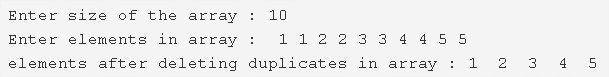# C Program To Delete Duplicate Elements From An Array | 4 Ways

C program to delete all duplicate elements from an array – In this article, we will specify the numerous ways to delete all duplicate elements from an array in C programming.

Suitable examples and sample programs have also been added so that you can understand the whole thing very clearly. The compiler has also been added with which you can execute it yourself.

The ways used here for C program delete duplicate elements from an array:

• Using Standard Method
• Using Function

Arrays are nothing but a collection of elements present in a sequential order horizontally. The arrays are a very significant part of C programming. The position of elements in an array is denoted with the help of pointers.As given in the image above, firstly, you need to enter the size of the given array.

The size of this particular array is 10.

Then, you need to enter the elements of that array.

The elements entered in this array are as follows:

1 1 2 2 3 3 4 4 5 5

As you can see, each element is repeated twice. Hence, after removing the duplicates, the array looks like this:

1 2 3 4 5

Thus, the means to the same in C programming are as follows:

## Using Standard Method

1. Read the array size and store it into the variable n.

2) Scanf reads the entered elements and stores the elements in the array using for loop for(i=0;i<n;i++).

3) To find duplicate elements in the array

for loop iterates from i=0 to i<n

a) if a[i]!=-1

compare each element of the string  with a[i] using for loop for(j=i+1;j<n;j++).if any element is equal to a[i] then increase the c value by 1 and put -1 in place of duplicate value i.e a[j]. Repeat until j<n.

b) If a[i]!=-1 place the a[i] into the array a[k++].Here we initializing the non-duplicate elements into the string a[k].

Repeat a,b steps until i<n.

4) Print the elements after deleting duplicates in array as

for loop iterates from i=0 to i<size of the array-number of duplicate elements

printf(“%d”,a[i]).

Output:

### Using Function

1. The main() function calls the count() function by passing array a, size of the array as arguments.

2) The count function finds the number of duplicate elements in the given array and returns the resultant array size to main() function as

for loop iterates from i=0 to i<array size

a) if a[i]!=-1

compare each element with a[i] using for loop for(j=i+1;j<n;j++).If any element match with a[i] then increase the count value by 1 and place -1 at a[j] which is duplicate element.Repeat until j<n.

b) If a[i]!=-1 store the a[i] into a[k++] for each iteration of i.

c) The count() function returns the n-c to the main() funtion and n-c initialized to n.

3) The main() function calls the print() function passing array,n as arguments.Then print() prints the elements after deleting duplicates in the array as printf(“%d”,a[i]) using for loop for(i=0;i<n;i++).

Output:

x

## C Program To Print Number Of Days In A Month | Java Tutoring

C program to input the month number and print the number of days in that ...